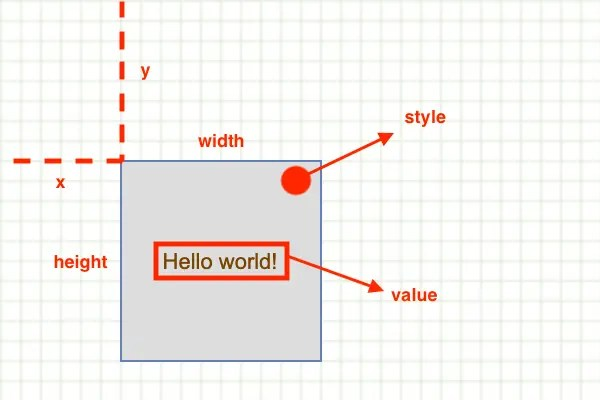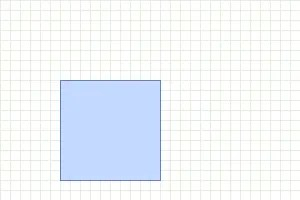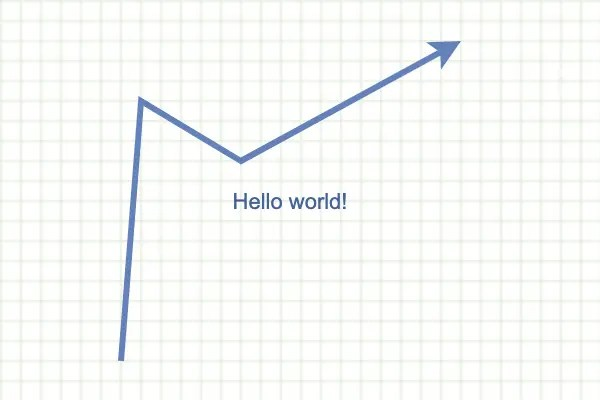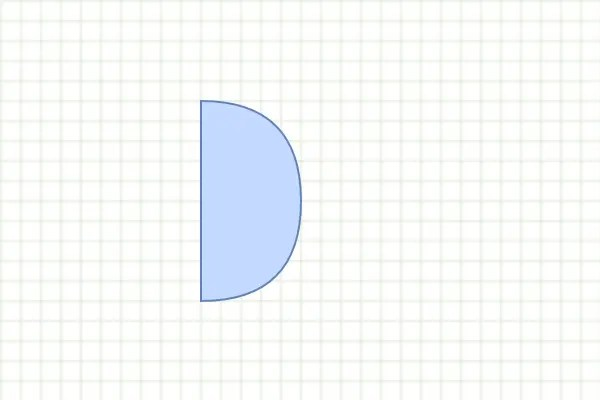# mxgraph series : bottom state tree mxCell

Posted May 25, 20209 min read

## 1 Introduction

mxgraph internally uses a tree-shaped data structure to record the content of graphic documents. In the content tree, each node represents a graphic element, and the element information is stored in the node On the object; the parent-child relationship of the node indicates that the parent node graph contains child node graphs, which can be used to realize the layering and grouping functions of the graph. For example, for the following graphic:

The content tree is the underlying data model for graphical documents, a bit like vdom to react; vnode to vue. Many functions of mxgraph are built around the content tree, such as the renderer mxCellRenderer according to the content tree Rendering graphic documents; codec mxCellCodec realizes the external storage format and content tree The conversion of each other; the basic operation units of various layout algorithms are also content tree nodes. Therefore, a deep understanding of the content tree model can lay a solid foundation for the source code learning process behind!

The content tree model is implemented by the following classes:

1. mxGraphModel :content tree model, mainly implements a series of tree structure operation methods.
2. mxCell :content tree node, used to store graphs, connecting lines, layering, etc. The status information of the primitive.
3. mxGeometry :the geometric information of the tree node, which records the width, height and coordinates of the graphic element Location; geometric characteristics such as the start node and end node of the connection.

The relationship of which class is above is as follows:

This article focuses on the usage of the content tree node mxCell , and the content about mxGraphModel and mxGeometry will be used later Special chapters are introduced.

## 2 . New to mxCell

mxCell class is defined in the `javascript/src/js/model/mxCell.js` file , Constructor signature:`function mxCell(value, geometry, style)`, parameter explanation:

• `value`:used to define the content of primitives, support to pass in dom objects and strings.
• `geometry`:the geometric value of the graph. For the vertex type, the x, y, width, and height attributes of the graph are recorded; the edge type also records the points connected at both ends of the line segment.
• `style`:graphic style

The following example shows how to construct an mxCell object:

``````//Construct mxCell instance
const cell = new mxCell('Hello world!', new mxGeometry(60, 80, 100, 100), 'fillColor = # ddd');
//Set cell to geometric pattern type
cell.vertex = true;

//Construct model using cell object
const model = new mxGraphModel(cell);
//Render the model
new mxGraph(document.getElementById('container'), model);``````

Rendering results:Tips:

The style function of mxgraph is extended based on the CSS style system. In addition to the standard style attributes, it also defines many own style attributes, which will be introduced in a separate chapter later.

## 3 . Drawing with mxCell

### 3.1 Rectangle

When `vertex = true`, the default graphic style of mxCell is a rectangle, so you only need to specify the starting coordinate and width and height attributes when rendering the rectangle, for example:

``````//Construct mxCell instance
const cell = new mxCell(
null,
//Specify the width, height, and starting coordinates of the rectangle through mxGeometry
new mxGeometry(60, 80, 100, 100)``````

);
//Set cell to geometric pattern type
cell.vertex = true;

Rendering effect:### 3.2 Line segment

The logic of using mxCell to draw line segments is slightly more complicated. Code snippet:

``````const cell = new mxCell('Hello world!', new mxGeometry(), 'strokeWidth = 3;');
//Set the cell as a line segment
cell.edge = true;
//Set the starting point
cell.geometry.setTerminalPoint(new mxPoint(60, 180), true);
//Set the endpoint
cell.geometry.setTerminalPoint(new mxPoint(230, 20), false);``````

The position of the line segment is determined by the end positions of the two ends of the line segment. For example, after initializing mxCell in the above example, you need to call `setTerminalPoint` to set the start and end positions of the line segment to render normally. The rendering result of the above example:Furthermore, you can use the `points` attribute to split multiple segments within a line segment, for example:

``````const cell = new mxCell('Hello world!', new mxGeometry(), 'strokeWidth = 3;');
//Set the cell as a line segment
cell.edge = true;
//Set the starting point
cell.geometry.setTerminalPoint(new mxPoint(60, 180), true);
//Set the endpoint
cell.geometry.setTerminalPoint(new mxPoint(230, 20), false);
//Use points to define multiple intermediate nodes
cell.geometry.points = [new mxPoint(70, 50), new mxPoint(120, 80)];``````

Rendering effect:### 3.3 More built-in graphics

In addition to rectangles and line segments, mxGraph also has built-in support for other graphics. Let s first look at an example:

``````<! DOCTYPE html>
<html lang = "zh-CN">
<meta charset = "utf-8" />
<meta http-equiv = "X-UA-Compatible" content = "IE = edge" />
<meta name = "viewport" content = "width = device-width, initial-scale = 1.0" />
<title> mxgraph Example </title>
<style>
#container {
background:url('../../assets/grid.gif');
}
</style>
<script type = "text/javascript">
mxBasePath = '//cdn.jsdelivr.net/npm/mxgraph@4.1.1/javascript/src';
</script>
<body>
<div id = "container" style = "width:400px; height:400px;"> </div>

<script src = "//cdn.jsdelivr.net/npm/mxgraph@4.1.1/javascript/mxClient.min.js"> </script>
<script type = "text/javascript">
function run(container) {
const shapes = [
//mxGraph has built-in support for the following graphics:
'actor',
'cloud',
'cylinder',
'doubleEllipse',
'ellipse',
'hexagon',
'image; image = https://jgraph.github.io/mxgraph/docs/images/mxgraph_logo.gif',
'rectangle',
'rhombus',
'swimlane',
'triangle',
];
const root = new mxCell(null, new mxGeometry(), null);
for(let i = 0; i <shapes.length; i ++) {
const shape = shapes [i];
const xOffset = i%4,
yOffset = Math.floor(i/4),
x = xOffset * 100 + 20,
y = yOffset * 100 + 20;
const geometry = new mxGeometry(x, y, 60, 60);
const cell = new mxCell(
shape.split(';') ,
geometry,
//Specify the cell's graphics category by shape
`shape = \${shape}; verticalLabelPosition = bottom; spacingBottom = 40`
);
cell.vertex = true;
root.insert(cell);
}
const model = new mxGraphModel(root);
new mxGraph(container, model);
}

run(document.getElementById('container'));
});
</script>
</body>
</html>``````

Example effect:In the example, when constructing the mxCell instance, you need to set the graphics category of the cell through the style parameter. The core code is:

``````const cell = new mxCell(
null,
new mxGeometry(0, 0, 100, 100),
//Modify shape category through shape attribute
`shape = triangle```````

);

If it is an image type, you also need to pass in the image address through `image`:

``````const cell = new mxCell(
null,
new mxGeometry(0, 0, 100, 100),
//shape attribute specifies bit image
//The image attribute sets the image address
`shape = image; image = https://jgraph.github.io/mxgraph/docs/images/mxgraph_logo.gif```````

);

### 3.4 Custom graphics

In mxGraph, the base class of all graphics is mxShape class, users only need to inherit Class can define a new graphics category, the core steps:

``````//1. Inherit mxShape class
class CustomShape extends mxShape {
constructor() {
super();
}

//Graphics rendering method
paintBackground(c, x, y, w, h) {}
}

//2. Register the graphics class in the renderer mxCellRenderer
mxCellRenderer.registerShape('customShape', CustomShape);

const cell = new mxCell(
null,
new mxGeometry(100, 50, 50, 100),
//3. When creating mxCell, the shape category is still defined by the shape attribute of the style parameter
'shape = customShape'``````

);

Complete example:

``````<! DOCTYPE html>
<html lang = "zh-CN">
<meta charset = "utf-8" />
<meta http-equiv = "X-UA-Compatible" content = "IE = edge" />
<meta name = "viewport" content = "width = device-width, initial-scale = 1.0" />
<title> mxgraph Example </title>
<style>
#container {
background:url('../../assets/grid.gif');
}
</style>
<!-Sets the basepath for the library if not in same directory->
<script type = "text/javascript">
mxBasePath = '//cdn.jsdelivr.net/npm/mxgraph@4.1.1/javascript/src';
</script>
<body>
<div id = "container" style = "width:300px; height:200px;"> </div>

<script src = "//cdn.jsdelivr.net/npm/mxgraph@4.1.1/javascript/mxClient.min.js"> </script>
<script type = "text/javascript">
//Inherit mxShape base class, extend custom graphics class
class CustomShape extends mxShape {
constructor() {
super();
}

paintBackground(c, x, y, w, h) {
c.translate(x, y);

c.ellipse(w/4, 0, w/2, h/4);
c.fillAndStroke();

c.begin();
c.moveTo(w/2, h/4);
c.lineTo(w/2,(2 * h)/3);

//Arms
c.moveTo(w/2, h/3);
c.lineTo(0, h/3);
c.moveTo(w/2, h/3);
c.lineTo(w, h/3);

//Legs
c.moveTo(w/2,(2 * h)/3);
c.lineTo(0, h);
c.moveTo(w/2,(2 * h)/3);
c.lineTo(w, h);
c.end();

c.stroke();
}
}

//Need to register graphics in the renderer mxCellRenderer
mxCellRenderer.registerShape('customShape', CustomShape);

function run(container) {
const cell = new mxCell(
'Hello world!',
new mxGeometry(100, 50, 50, 100),
//Still specify the graphics category through the shape attribute of the style parameter
'shape = customShape'
);
cell.vertex = true;

const model = new mxGraphModel(cell);
new mxGraph(container, model);
}

run(document.getElementById('container'));
});
</script>
</body>
</html>``````

Example effect:### 3.5 Drawing with stencils

In addition to implementing custom graphics classes by extending `mxShape`, you can also use the stencils interface to define new graphics categories. The main steps are:

``````//1. Define the graphic content in xml format
const shapes = `<shapes> ... </shapes>`;
//2. Convert the string to a DOM object
const node = new DOMParser(). parseFromString(shapes, 'text/xml');
//3. Register stencils object

const cell = new mxCell(
null,
new mxGeometry(100, 50, 50, 100),
//4. When creating mxCell, the shape category is still defined by the shape attribute of the style parameter
'shape = or'``````

);

Complete example:

``````<! DOCTYPE html>
<html lang = "zh-CN">
<meta charset = "utf-8" />
<meta http-equiv = "X-UA-Compatible" content = "IE = edge" />
<meta name = "viewport" content = "width = device-width, initial-scale = 1.0" />
<title> mxgraph Example </title>
<style>
#container {
background:url('../../assets/grid.gif');
}
</style>
<!-Sets the basepath for the library if not in same directory->
<script type = "text/javascript">
mxBasePath = '//cdn.jsdelivr.net/npm/mxgraph@4.1.1/javascript/src';
</script>
<body>
<div id = "container" style = "width:300px; height:200px;"> </div>

<script src = "//cdn.jsdelivr.net/npm/mxgraph@4.1.1/javascript/mxClient.min.js"> </script>
<script type = "text/javascript">
//xml format defines the graphic content
const shapes = `<shapes>
<shape name = "or" aspect = "variable">
<background>
<path>
<move x = "0" y = "0" />
<quad x1 = "100" y1 = "0" x2 = "100" y2 = "50" />
<quad x1 = "100" y1 = "100" x2 = "0" y2 = "100" />
<close />
</path>
</background>
<foreground>
<fillstroke />
</foreground>
</shape>
</shapes> `;
//Parse the XML value of the string into a dom object
const parser = new DOMParser();
const node = parser.parseFromString(shapes, 'text/xml');
//Register brush

function run(container) {
const cell = new mxCell(null, new mxGeometry(100, 50, 50, 100), 'shape = or');
cell.vertex = true;

const model = new mxGraphModel(cell);
new mxGraph(container, model);
}

run(document.getElementById('container'));
});
</script>
</body>
</html>``````

Example effect:Tips:

Custom mxShape and stencils interfaces can be extended to new graphics categories, mxShape is defined by class form, there will be a certain development cost, but more graphics logic can be customized; and stencils can be defined, developed and managed by external xml files It will be more convenient. The specific method used can be selected according to actual needs.

Both sets of methodologies are more complicated, and the author will not be able to make it clear in a small segment. The author will open a special topic later to give a detailed introduction.

<!-TODO->
<!-Violated the single principle->

<!-TODO->
<!-Section 4->
<!-How to perform transformation, for example:translate, rotate, etc.->

## 4 . Summary

So far, this section is almost over. The article mainly introduces the underlying data structure mxCell and how to use mxCell to draw a variety of different graphics. With the deepening of learning, we found that there are more points that can be tapped, including:

1. How to use mxGraphModel
2. How to use and operate the style system in mxGraph
3. How to perform geometric transformation of graphics
4. How to use custom graphics and custom stencils
5. ...

In the future, we will gradually supplement a series of articles, and strive to help people in need to understand the usage and principles of mxGraph in depth. Interested students are welcome to pay attention.